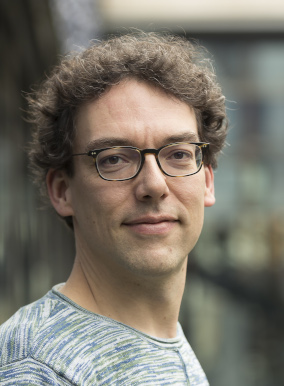# Biostatistics Weekly Seminar

## Towards Markov Properties for Differential-Algebraic EquationsJoris M. Mooij, PhDProfessor in Mathematical Statistics Korteweg-De Vries Institute for Mathematics University of AmsterdamReal-world systems---both static and dynamic---are often modelled by differential-algebraic equations (DAEs), systems of equations in which first and higher-order derivatives may appear. Well-known examples are chemical reactions, electric circuits and constrained mechanical systems. DAEs generalize systems of (ordinary, algebraic) equations as well as ordinary differential equations. Stochasticity can be introduced via exogenous variables and initial states. However, DAEs have no intrinsic notion of causality, unlike for example structural (dynamical) causal models. We show how the structure of the equations nonetheless gives rise to Markov properties by means of Simon's causal ordering algorithm and the Dulmage-Mendelsohn decomposition, thereby extending well-known Markov properties for directed graphical models to DAEs represented by bipartite graphs. As an immediate consequence, we obtain possible causal interpretations of the DAE as well as formal causal reasoning methods for domain adaptation that extend Pearl's do-calculus. Our approach also provides the building blocks towards generalizing identification methods (such as Tian's ID algorithm) and constraint-based causal discovery (such as the FCI algorithm) towards a very general class of (possibly) cyclic and (possibly) dynamical systems.

### Zoom (Link to Follow)30 November 20221:30pm

Speaker Itinerary

Topic revision: r1 - 18 Nov 2022, CierraStreeter

• Biostatistics WebsCopyright © 2013-2022 by the contributing authors. All material on this collaboration platform is the property of the contributing authors.
Ideas, requests, problems regarding Vanderbilt Biostatistics Wiki? Send feedback